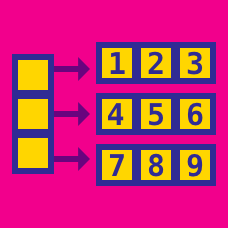Computer Science

# Arrays - Intermediate

Given a 2D $N\times M$ matrix print it in spiral form. That is start from the top left corner and traverse clockwise.

Example

$\begin{bmatrix} 3 & 5& 4& 1\\ 4& 10& 9 &2 \\ 5& 2& 8 &13 \\ 6& 1& 7& 18 \end{bmatrix}$

When the above code is implemented it outputs 3, 5, 4, 1, 2, 13, 18, 7, 1, 6, 5, 4, 10, 9, 8, 2

What will the above function output when the following Matrix is inputted.

$\begin{bmatrix} 1& 2& 11& 4& 6\\ 9& 2& 7& 8& 11\\ 3& 1& 13& 12 & 2\\ 8& 14& 11& 16& 4\\ 17& 19 & 17 & 7 & 8 \end{bmatrix}$

Given a 2D square Array,write a method that finds the product of all elements in column $x$. Also write a method that returns the sum of all elements in row $x$.

In the $10 \times 10$ matrix below, let $S$ be the sum of all column products and let $T$ be the sum of all row sums. What are the last three digits of $S + T$?

  1 2 3 4 5 6 7 8 9 10 {5, 10, 10, 1, 6, 4, 3, 9, 6, 4}, {2, 10, 9, 4, 9, 5, 1, 10, 1, 5}, {1, 3, 7, 3, 10, 7, 5, 1, 7, 5}, {5, 1, 2, 7, 3, 2, 4, 2, 1, 3}, {7, 6, 6, 6, 4, 10, 5, 1, 5, 5}, {3, 1, 10, 5, 8, 1, 9, 10, 2, 7}, {1, 7, 1, 10, 5, 10, 5, 3, 3, 3}, {6, 3, 10, 2, 5, 1, 6, 7, 10, 9}, {1, 7, 9, 6, 2, 7, 10, 1, 9, 6}, {10, 9, 6, 10, 4, 7, 6, 3, 4, 7} 

Given an array and a number $t$, write a function that determines if there exists a contiguous sub-array whose sum is $t$.

Which of the below arrays contains a sub-array whose sum is $316$?

A. {61, 6, 39, 29, 30, 72, 98, 36, 42, 66, 24, 58, 13, 16, 73}

B. {87, 78, 4, 10, 48, 43, 33, 70, 21, 18, 75, 66, 39, 80, 87}

C. {82, 72, 39, 67, 65, 93, 28, 2, 89, 39, 68, 29, 61, 14, 98}

D. {45, 5, 14, 75, 100, 37, 98, 64, 90, 52, 66, 30, 18, 89, 19}

×